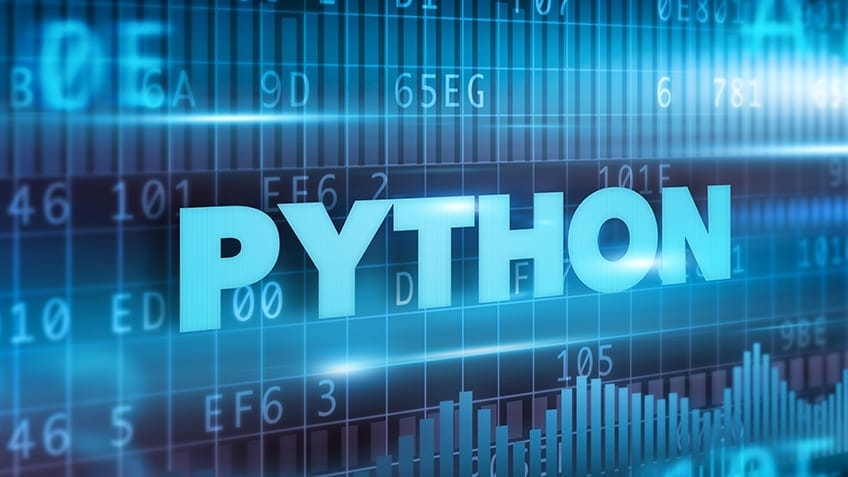Numbers in Python refer to the numeric data types in Python programming. Python supports three kinds of numeric data types: int, float, and complex. In this tutorial, we’ll learn how to use numbers in Python programming. We’ll use Jupyter Notebook to implement the code.

Looking forward to make a move to programming? Take up the Python Training Course and begin your career as a professional Python programmer.

Let’s see how to use numbers and what their significance is their significance in programming.

Integers are numbers without decimal points.

num=5

type(num)

num=23475891

type(num)Floats are numbers with decimal points.

num=5.4

type(num)Complex numbers have real parts and imaginary parts.

num=2+5j

type(num)

num.real #Gives the real part of the complex number

num.imag #Gives the imaginary part of the complex numberComplex numbers store the real and imaginary parts as a float by default.

Numbers can also be negative, which you can store in a variable.

num=-423.31

print(num)Performing arithmetic operations using numbers in Python is simple and easy to understand. The following examples will help you understand how to add, subtract, multiply and divide numbers in Python:

num1=10

num2=2

print(num1+num2)

print(num1-num2)

print(num1*num2)

print(num1/num2)In Python, the output of any division problem is a floating-point value. To receive the output as an integer, use the integer division//’.

print(10/3)

print(10//3)If you want to raise the power of a number to a certain value, use the double asterisks (**).

print(num1**num2) #This will print 10 raised to the power two.

In case you want to get the remainder instead of the quotient, use the modulo operator.

print(num1%num2)

print(10%3)Next, let’s see how to convert the data type of a variable into another data type using type conversion techniques.

In this example, x has a value of 192. Since it is stored within quotes, it is a string value.

x=”192”

type(x)

To convert it into an integer, you need to use the int() function.Similarly, to convert the value of x into a float, use the float() function.

x=float(x)

print(x)

The following line of code will convert x to a complex number:

x=complex(x)

print(x)There is another way to generate complex numbers in Python. Use the complex function and pass the parameters as real and imaginary.

Syntax: complex(real, imaginary)print(complex(2,6)

Next, we will demonstrate how to use Python built-in functions using numbers.

A number can be either positive or negative. If you only want to get the positive value of a number, use the absolute function abs().

x = -7.5

print(abs(x))In Python, you can calculate the exponent of a number. To do this, you need to import the math module.

import math

x=10

print(math.exp(x))

If you are wondering what the value of e is, use “math.e”. Python holds “e” as a constant.You can print the value of pi as follows:

print(math.pi)

Python has other functions in the math module, such as sqrt(), which provides the square root of a number.

print(math.sqrt(4))

print(math.sqrt(6))Python provides other built-in functions, such as max() and min(), to perform operations on numbers.

print(max(34,475,32,278)) #Returns the maximum value

print(min(34,475,32,278)) #Return the minimum valueLet’s write a program to check if a number is prime or not:

n=input("Enter a number ")

n=int(n)

flag=0

for i in range(2,n):

if n%i==0:

flag=1

print("%d is not a prime number"%n)

break

if flag==0:

print("%d is a prime number"%n)In the following example, you will see how to find the solution to a quadratic equation. A quadratic equation is of the form a*x2 + b*x + c. The user needs to enter the values for a, b, and c. You also need the value of the discriminant d. The formula for d is b2-4*a*c.As you can see, the roots from the given inputs are incorrect since Python evaluates expressions using the BODMAS rule. You will need to change the else part of the program and use brackets in the appropriate places.Are you interested to create Learn to create your own Python programs? Check out the Python Training Course. Click to enroll now!

## Conclusion

I hope this blog has helped you learn about numbers in Python, including which ones are supported. You learned how to perform arithmetic operations on Python, and how type conversion works. You came across some important built-in functions used in Python. Finally, you learned to write Python programs to check if a number is prime or not, all while finding the solution to a quadratic equation. To learn more about numbers in Python, watch this video:Avijeet Biswal